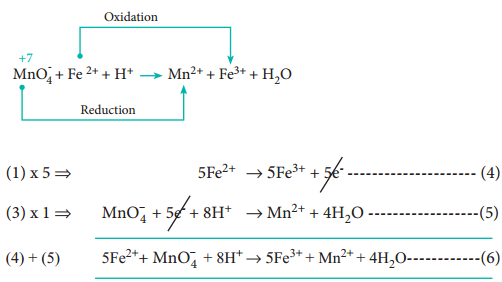Find free online Chemistry Topics covering a broad range of concepts from research institutes around the world.

## Oxidation Number Defination and Examples

It is defined as the imaginary charge left on the atom when all other atoms of the compound have been removed in their usual oxidation states that are assigned according to set of rules. A term that is often used interchangeably with oxidation number is oxidation state.

1. The oxidation state of a free element (i.e. in its uncombined state) is zero.
Example: each atom in H2, Cl2, Na, S8 have the oxidation number of zero.

2. For a monatomic ion, the oxidation state is equal to the net charge on the ion.

Example:

The oxidation number of sodium in Na+ is +1.

The oxidation number of chlorine in Cl is -1.

3. The algebric sum of oxidation states of all atoms in a molecule is equal to zero, while in ions, it is equal to the net charge on the ion.

Example:

In H2SO4; 2 × (oxidation number of hydrogen) + (oxidation number of S) + 4 (oxidation number of oxygen) = 0.

In SO42-; (oxidation number of S) + 4 (oxidation number of oxygen) = – 2.

4. Hydrogen has an oxidation number of +1 in all its compounds except in metal hydrides where it has – 1 value.

Example:

Oxidation number of hydrogen in hydrogen chloride (HCl) is + 1.
Oxidation number of hydrogen in sodium hydride (NaH) is -1.

5. Fluorine has an oxidation state of – 1 in all its compounds.

6. The oxidation state of oxygen in most compounds is – 2. Exceptions are peroxides, super oxides and compounds with fluorine.

Example:

Oxidation number of oxygen,

(i) in water (H2O) is – 2.

(ii) in hydrogen peroxide (H2O2) is -1.
2 (+ 1) + 2x = 0;
⇒ 2x = – 2;
⇒ x = – 1

(iii) in super oxides such as KO2 is – 1
+1 + 2x = 0;
2x = – 1;
x = – 1/2

(iv) in oxygen difloride (OF2) is + 2.
x + 2 (- 1) = 0;
x = + 2

7. Alkali metals have an oxidation state of + 1 and alkaline earth metals have an oxidation state of + 2 in all their compounds.

Calculation of Oxidation Number Using the Above Rules.Redox Reactions in Terms of Oxidation Numbers

During redox reactions, the oxidation number of elements changes. A reaction in which oxidation number of the element increases is called oxidation. A reaction in which it decreases is called reduction.

Consider the following reaction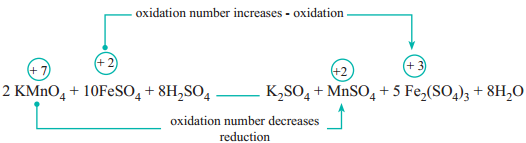In this reaction, manganese in potassium permanganate (KMnO4) favours the oxidation of ferrous sulphate (FeSO4) into ferric sulphate (Fe2(SO4)3 by gaining electrons and thereby gets reduced. Such reagents are called oxidising agents or oxidants. Similarly, the reagents which facilitate reduction by releasing electrons and get oxidised are called reducing agents.

Types of Redox Reactions

Redox reactions are classified into the following types.

1. Combination Reactions:

Redox reactions in which two substances combine to form a single compound are called combination reaction.

Example: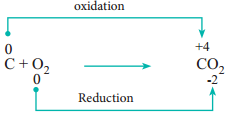2. Decomposition Reactions:

Redox reactions in which a compound breaks down into two or more components are called decomposition reactions. These reactions are opposite to combination reactions. In these reactions, the oxidation number of the different elements in the same substance is changed.

Example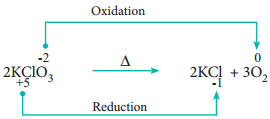3. Displacement Reactions:

Redox reactions in which an ion (or an atom) in a compound is replaced by an ion (or atom) of another element are called displacement reactions. They are further classified into

• Metal Displacement Reactions
• Non-Metal Displacement Reactions

Metal Displacement Reactions:

Place a zinc metal strip in an aqueous copper sulphate solution taken in a beaker. Observe the solution, the intensity of blue colour of the solution slowly reduced and finally disappeared. The zinc metal strip became coated with brownish metallic copper. This is due to the following metal displacement reaction.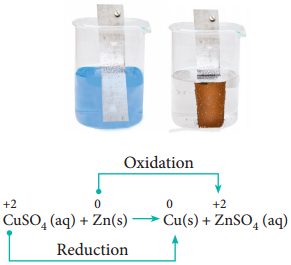Non-Metal Displacement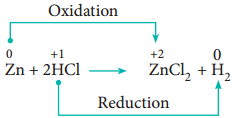4. Disproportionation Reaction (Auto Redox Reactions)

In some redox reactions, the same compound can undergo both oxidation and reduction. In such reactions, the oxidation state of one and the same element is both increased and decreased. These reactions are called disproportionation reactions.

Examples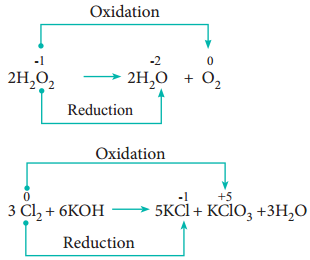5. Competitive Electron Transfer Reaction

In metal displacement reactions, we learnt that zinc replaces copper from copper sulphate solution. Let us examine whether the reverse reaction takes place or not. As discussed earlier, place a metallic copper strip in zinc sulphate solution. If copper replaces zinc from zinc sulphate solution, Cu2+ ions would be released into the solution and the colour of the solution would change to blue.

But no such change is observed. Therefore, we conclude that among zinc and copper, zinc has more tendency to release electrons and copper to accept the electrons.

Let us extend the reaction to copper metal and silver nitrate solution. Place strip of metallic copper in sliver nitrate solution taken in a beaker. After some time, the solution slowly turns blue. This is due to the formation of Cu2+ ions, i.e. copper replaces silver from silver nitrate.

The reaction is,It indicates that between copper and silver, copper has the tendency to release electrons and silver to accept electrons.

From the above experimental observations, we can conclude that among the three metals, namely, zinc, copper and silver, the electron releasing tendency is in the following order.

Zinc > Copper > Silver

This kind of competition for electrons among various metals helps us to design (galvanic) cells. In XII standard we will study the galvanic cell in detail.

Balancing (the Equation) of Redox Reactions

The two methods for balancing the equation of redox reactions are as follows.

• The oxidation number method
• Ion-electron method / half reaction method.

Both are based on the same principle:

In oxidation – reduction reactions the total number of electrons donated by the reducing agent is equal to the total number of electrons gained by
the oxidising agent.

Oxidation Number Method

In this method, the number of electrons lost or gained in the reaction is calculated from the oxidation numbers of elements before and after the reaction. Let us consider the oxidation of ferrous sulphate by potassium permanganate in acid medium. The unbalanced chemical equation is,

FeSO4 + KMnO4 + H2SO4 → Fe2(SO4)3 + MnSO4 + K2SO4 + H2O

Step 1

Using oxidation number concept, identify the reactants (atom) which undergo oxidation and reduction.(a) The oxidation number of Mn in KMnO4 changes from +7 to +2 by gaining five electrons.

(b) The oxidation number of Fe in FeSO4 changes from +2 to +3 by loosing one electron.

Step 2

Since, the total number of electrons lost is equal to the total number of electrons gained, equate, the number of electrons, by cross multiplication of the respective formula with suitable integers on reactant side as below. Here, the product Fe2(SO4)3 contains 2 moles of iron, So, the Coefficients 1e & 5e are multiplied by the number ‘2’.10 FeSO4 + 2 KMnO4 + H2SO4 → Fe2(SO4)3 + MnSO4 + K2SO4 + H2O

Step 3

Balance the reactant / Product Oxidised / Reduced

Now, based on the reactant side, balance the products (ie oxidised and reduced).The above equation becomes

10FeSO4 + 2KMnO4 + H2SO4 → 5Fe2(SO4)3 + 2MnSO4 + K2SO4 + H2O

Step 4

Balance the other elements except H and O atoms. In this case, we have to balance K and S atoms but K is balanced automatically.

Reactant Side:

10 ‘S’ atoms (10 FeSO4)

Product Side:

18 ‘S’ atoms

5Fe2(SO4)3 + 2MnSO4 + K2SO4

15S + 2S + 1S = 18S

Therefore the difference 8-S atoms in reactant side, has to be balanced by multiplying H2SO4 by ‘8’ The equation now becomes,

10FeSO4 + 2KMnO4 + 8H2SO4 → 5Fe2(SO4)3 + 2MnSO4 + K2SO4 + H2O

Step 5

Balancing ‘H’ and ‘O’ atoms

Reactant side ’16’-H atoms (8H2SO4 i.e. 8 × 2H = 16 ‘H’)

Product side ‘2’ – H atoms (H2O i.e. 1 × 2H = 2 ‘H’)

Therefore, multiply H2O molecules in the product side by ‘8’

10 FeSO4 + 2 KMnO4 + 8 H2SO4 → 5 Fe2(SO4)3 + 2 MnSO4 + k2SO4 + 8H2O

The oxygen atom is automatically balanced. This is the balanced equation.

Ion – Electron Method

This method is used for ionic redox reactions.

Step 1

Using oxidation number concept, find out the reactants which undergo oxidation and reduction.

Step 2

Write two separate half equations for oxidation and reduction reaction, Let us consider the same example which we have already discussed in
oxidation number method.

KMnO4 + FeSO4 + H2SO4 → MnSO4 + Fe2(SO4)3 + K2SO4 + H2O

The ionic form of this reaction is,

The two half reactions are,

Fe2+ → Fe3+ + 1e ………………….. (1)
and
MnO4 + 5e → Mn2+ …………………. (2)

Balance the atoms and charges on both sides of the half reactions.

Equation (1) ⇒ No changes i.e.,

Fe2+ → Fe3+ + 1e ………………….. (1)

Equation (2) ⇒ 4’O’ on the reactant side, therefore add 4H2O on the product side, to balance ‘H’ – add, 8H+ in the reactant side

MnO4 + 5e + 8H+ → Mn2+ + 4H2O ……………… (3)

Step 3

Equate both half reactions such that the number of electrons lost is equal to number of electrons gained. Addition of two half reactions gives the balanced equation represented by equation (6).